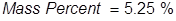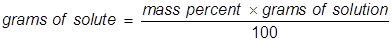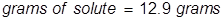# Mass Percent Formula

Mass Percent Formula

Mass percent is used as a way of expressing a concentration or a way of describing a component in a mixture. For a solution, the mass percent is expressed as the grams of solute divided by the grams of solution, then multiplied by 100 to get a percentage.Mass Percent Formula Questions:

1. Calculate the mass percent of sodium hypochlorite in commercial bleach, if 1.00 grams of NaOCl (the active ingredient in bleach) is dissolved in 19.05 grams of solution.

Answer:2. Calculate the grams of NaOCl (5.25% by mass) in 245 grams of a commercial bleach solution.

Answer:

In this problem the equation should be rearranged to solve for the grams of solute.Related Links: Force, Mass, Acceleration Quiz Mass vs. Weight